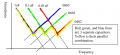# Purpose of the Capacitor in this filter

#### ericgibbs

Joined Jan 29, 2010
17,433
Hi sitheesh,
Various types of capacitor construction have different frequency versus impedance characteristics.
They are paralleled to give a wider bandwidth range for the filter.
E

•StefanZe

#### crutschow

Joined Mar 14, 2008
32,064
For the simulation there is no purpose, since all the capacitors are ideal.

For the real circuit there is, as Eric noted.

#### MrChips

Joined Oct 2, 2009
28,517
Are you sure the pi-filter is made up of C5, L3, C6?

C8 = 22pF
C7 = 100000pF
C5 = 10000pF

Altogether, these three capacitors in parallel would add up to 110,022pF.
The tolerance of C7 alone could be 10% or 20%. What effect would C5 and C8 have on the overall capacitance?

This graph should provide you with some insight as to why we put different values of decoupling capacitors in parallel.•ronsimpson

#### Ian0

Joined Aug 7, 2020
7,475
Have a look at this datasheet:
https://docs.rs-online.com/d3ac/0900766b802d4698.pdf
Look at the frequency vs. impedance graphs, and determine the frequency at the lowest impedance. It is the self-resonant frequency.
Then calculate L=1/(4.π^2.f^2.C), and put that value of inductance in series with the capacitor in SPICE.
And put a value of resistor equivalent to the minimum impedance in series with the L and C.
Then you have a rough approximation to a "real" capacitor.
Do that with all four capacitors.
Then run the simulation.
(There's also a leakage resistance that should go in parallel with the combination of R, L and C, but it is so large that it will rarely make any difference)

#### sitheesh

Joined Jan 3, 2023
9
Have a look at this datasheet:
https://docs.rs-online.com/d3ac/0900766b802d4698.pdf
Look at the frequency vs. impedance graphs, and determine the frequency at the lowest impedance. It is the self-resonant frequency.
Then calculate L=1/(4.π^2.f^2.C), and put that value of inductance in series with the capacitor in SPICE.
And put a value of resistor equivalent to the minimum impedance in series with the L and C.
Then you have a rough approximation to a "real" capacitor.
Do that with all four capacitors.
Then run the simulation.
(There's also a leakage resistance that should go in parallel with the combination of R, L and C, but it is so large that it will rarely make any difference)
Hi Ian, Thanks for the explanation. For this 06035C104KAT2A there is no graphs mentioned. So if this parallel capacitor is for having a wide bandwidth, why it is not placed symmetrically (only in parallel to the first capacitor side).

#### Ian0

Joined Aug 7, 2020
7,475
Hi Ian, Thanks for the explanation. For this 06035C104KAT2A there is no graphs mentioned. So if this parallel capacitor is for having a wideπ bandwidth, why it is not placed symmetrically (only in parallel to the first capacitor side).
It may depend on the purpose of the filter. It is to stop interference from the supply getting into the circuit or is it to stop interference from the circuit getting into the supply?
By the way, your simulation won’t work for a π-section filter because a π-section filter is for a situation with a non-zero sourceimpedance.
For a low source impedance, a T-section filter is required.
Either that, or put an appropriate source impedance in series with your source.
(The UK mains supply has a source impedance of about 0.4+0.25jΩ, which might be a useful starting point, if that is what you are trying to simulate.)

#### MrChips

Joined Oct 2, 2009
28,517
RF noise can enter a circuit by any means possible.
There is no difference between a positive supply line or negative supply line.

#### StefanZe

Joined Nov 6, 2019
158
This can be used to seperate the ground signals for high frequency noise (this often creates more problems than it solves).

•nsaspook